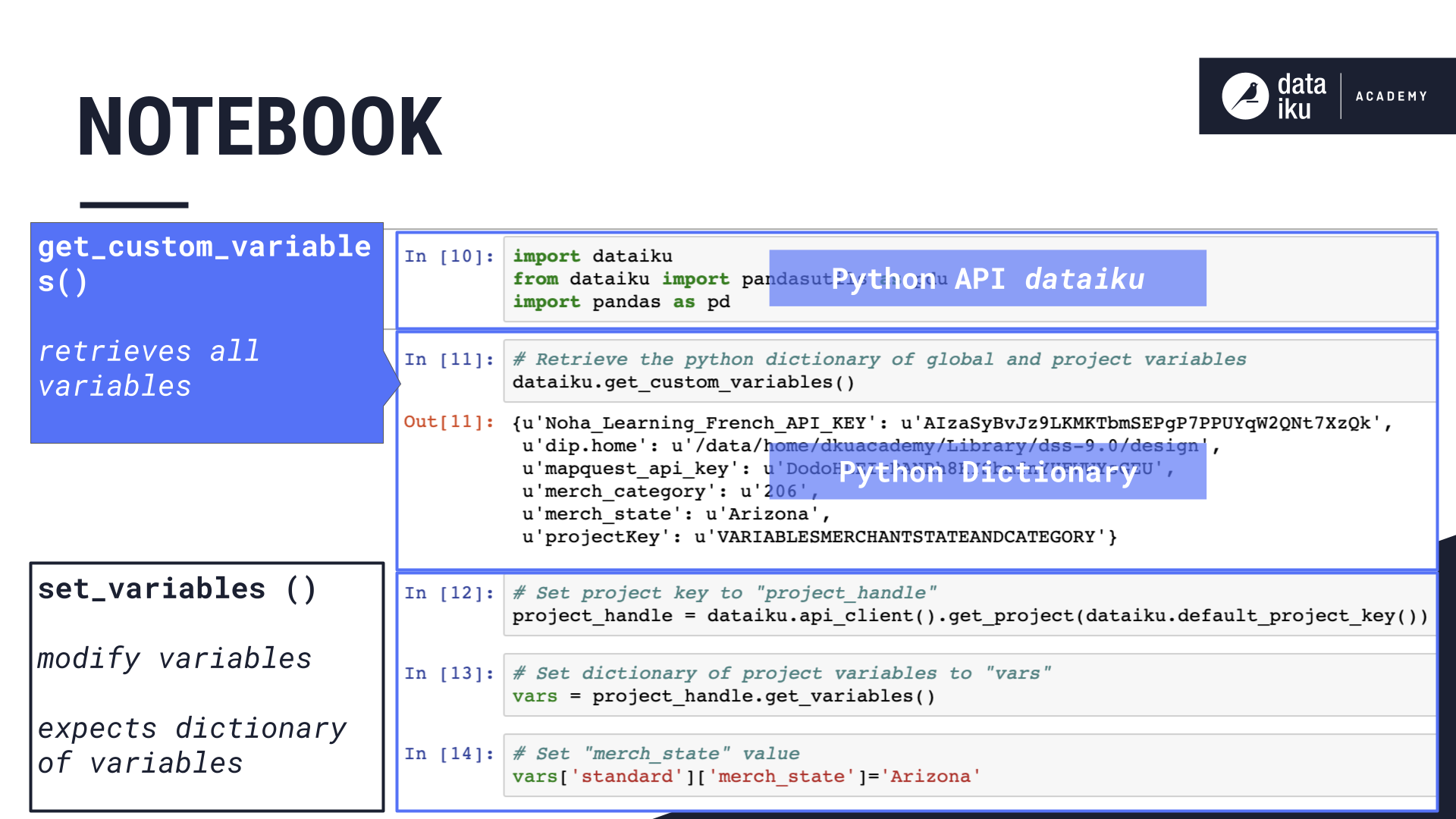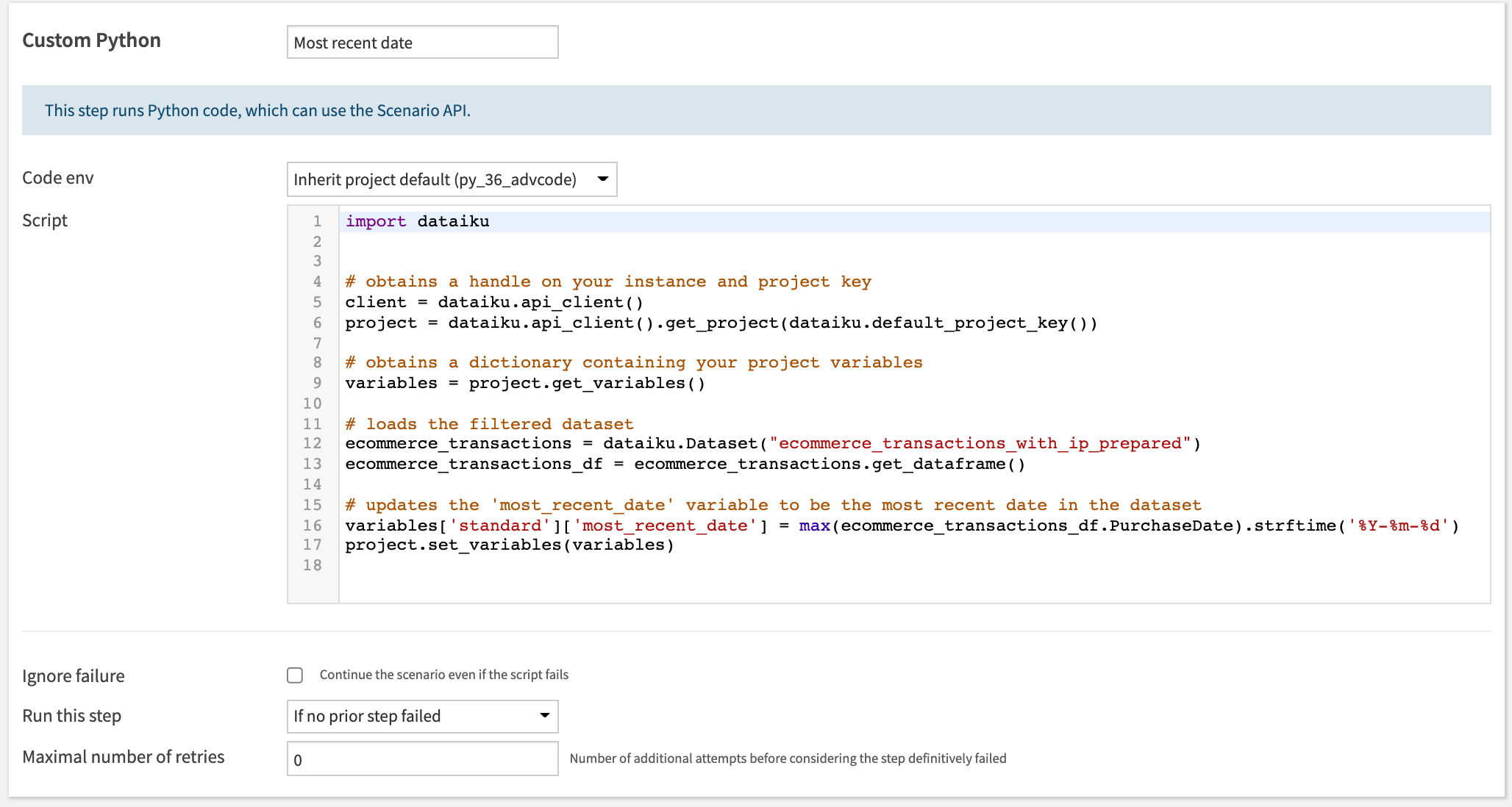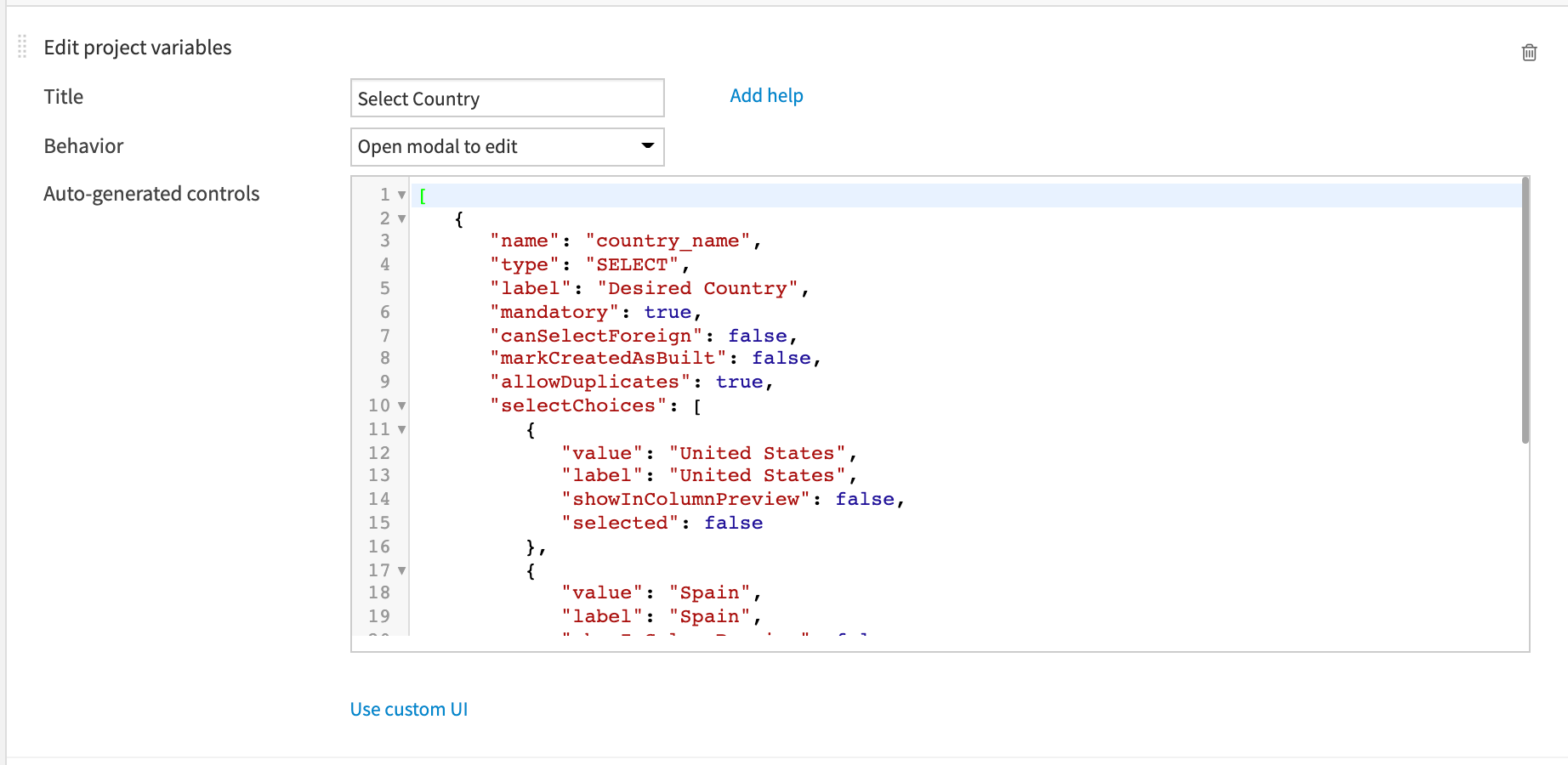# Concept Summary: Modifying the Value of Variables¶

In this lesson, we’ll discuss three ways we can modify the value of our variables using code including: a Jupyter notebook, executing python code in a scenario, and creating a Dataiku application that executes code.

Note

Changing the instance-level global variables is not recommended.

One way to change the value of our variables would be to manually edit the value where we defined our variables on the Project variables page.

However this is not always practical.

## Jupyter Notebook¶

A more practical solution is to modify the value of our variables using code.

Let’s say we want to change the value of our “merch_state” variable from “California” to “Arizona”.

When working with variables in a Python recipe, recall that we are using the Python API package, “dataiku”, and that this package includes a Python dictionary.

To modify the value of our variable, we’ll be working with two main functions, one to “Get” our variables, and one to “Set” them.

The “Get Custom Variables” function allows us to retrieve all the global and project variables that are stored in the Python dictionary. While the “Set Variables” function allows us to modify them.

When using “Set Variables”, Dataiku DSS expects the entire dictionary of project variables to be included. But we want to modify just one variable.

To do this, we’ll start by creating a new Python notebook where the package, dataiku, is already imported for us. Then we’ll use Get Custom Variables to determine what the current values of our variables are. We’ll then set the value of our default project key to a variable called “project handle”.

Note

The default project key is the same as the key for the current project.

Then we’ll store the dictionary of project variables in an object called “vars”. Now we can update the value of our “merch_state” variable to “Arizona”. And finally, we’ll update the variables in our python dictionary using the Set Variables function.

Now when we Get Custom Variables again we can see that the value of “merch_state” has been updated.Note

We can always print the value of a specific variable using the variable’s key.

We can then create a Python recipe from our notebook, thereby adding a new recipe to the Flow.

## Scenario¶

Another way to modify the value of a variable is to use a scenario.

Using a scenario, we can add a step to execute Python code to modify the value of our variable.

For example, we can create a scenario with a step where we write Python code to update the “most recent date” variable to be the most recent date in the dataset.

The main difference is that in our step, we will manually import the dataiku package, because it is not automatically done for us.## Dataiku Application¶

Finally, another way to modify the value of a variable is through a reusable Dataiku application. In this way, we can allow project collaborators who are non-coders to update project variables.

For example, this application allows the user to select the value of “merch_state”.

The application design uses a special tile called Edit project variables which provides starter code that you can customise.## What’s Next?¶

In this lesson, we learned about modifying the value of our variables using a Jupyter notebook, a scenario, and a Dataiku application. To go further, check out the other lessons in this course!